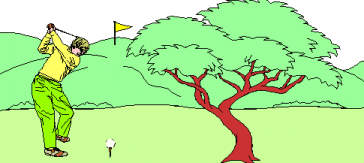Teaching Physics with the Physics Suite Edward F. Redish

Hitting the green

A golfer is trying to hit a golf ball onto the green. The green is a horizontal distance s from his tee and it is up on the side of a hill a height h above his tee. When he strikes the ball it leaves the tee at an angle q to the horizontal. He wants to know with what speed, v0, the ball must leave the tee in order to reach the height h at the distance s.1. Once he has struck the ball, what controls its motion? Write the equations that determine the vector acceleration of the golf ball after it leaves the tee. Be sure to specify your coordinate system. For this part of the problem you may ignore air resistance.
2. Solve the equations you have written in (a) to obtain expressions which can be evaluated to give the position of the ball at any time, t.
3. If the golfer wants his ball to land in the right place, he must hit it so that it leaves the tee with the right speed. Explain how he can calculate it. (Again, you may ignore air resistance.) Find an equation for the initial speed in terms of the problem's givens.
4. If the ball leaves the tee at an angle of 30ş, s = 100 m, and h = 10 m, find the speed with which the ball should leave the tee.
5. Now consider the effect of air resistance. Suppose that a good model for the force of air resistance is the Newton drag law,where |v| stands for the absolute value of the velocity -- the speed. Consider three points of the ball's trajectory -- halfway up, at its highest point, and halfway down. Discuss the direction of the resistance force at each place. Qualitatively (do not attempt a calculation!), what will the effect of air resistance be on the ball's motion?

Not finding what you wanted? Check the Site Map for more information.